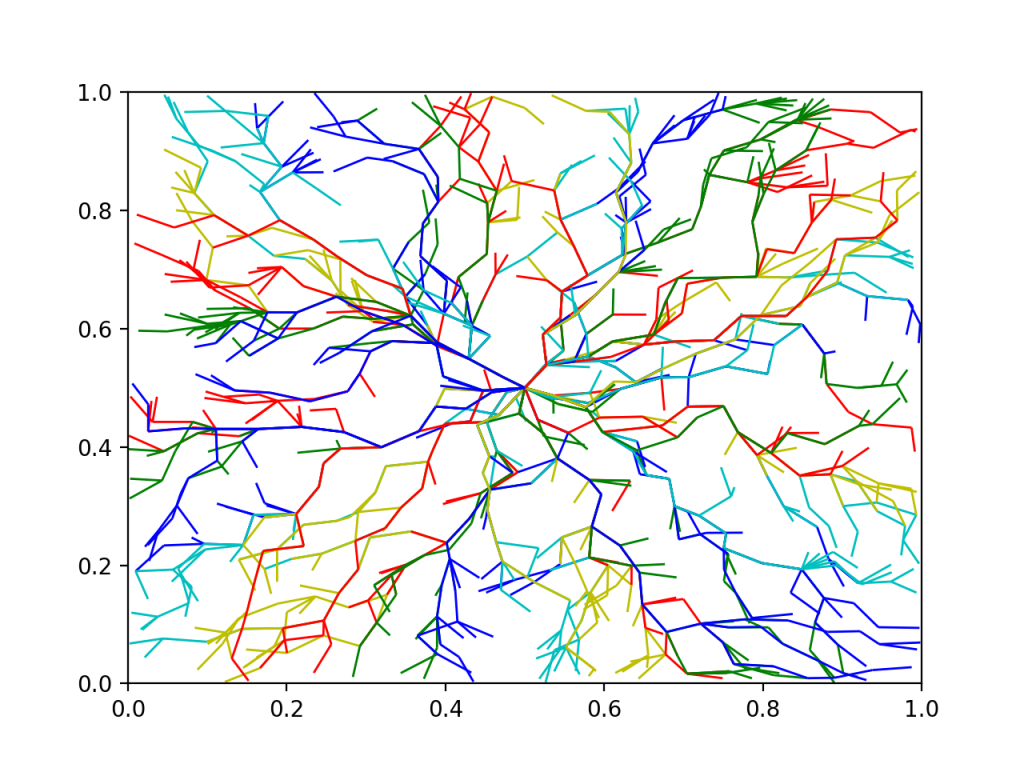Networkx outputs scipy sparse incidence matrices

https://networkx.github.io/documentation/networkx-1.9/reference/generated/networkx.linalg.graphmatrix.incidence_matrix.html#networkx.linalg.graphmatrix.incidence_matrix

https://docs.scipy.org/doc/scipy/reference/sparse.html

Networkx also has it’s own flow solvers, but cvxpy gives you some interesting flexibility, like turning the problem mixed integer, quadratic terms, and other goodies. Plus it is very easy to get going as you’ll see.

So here’s a basic example of putting these two together. Very straightforward and cool.



import networkx as nx
import cvxpy as cvx
import matplotlib.pyplot as plt
import numpy as np
from scipy.sparse import lil_matrix

#graph is an networkx graph from somewhere

#print(edgedict)
nEdges = len(graph.edges)
nNodes = len(graph.nodes)

posflow = cvx.Variable(nEdges)
negflow = cvx.Variable(nEdges)

# split flow into positive and negative parts so we can talk about absolute value.
# Perhaps I should let cvxpy do it for me
constraints = [ 0 <= posflow,  0 <= negflow ]

absflow = posflow + negflow
flow = posflow - negflow

L = nx.incidence_matrix(graph, oriented=True )

source = np.zeros(nNodes) #lil_matrix(n_nodes)
# just some random source placement.
source = 1
source = -1

# cvxpy needs sparse matrices wrapped.
Lcvx = cvx.Constant(L)
#sourcecvx = cvx.Constant(source)

# flow conservation
constraints.append(Lcvx*flow == source)

# can put other funky inequality constraints on things.

objective = cvx.Minimize(cvx.sum(absflow))

print("building problem")
prob = cvx.Problem(objective, constraints)
print("starting solve")
prob.solve(solver=cvx.OSQP, verbose = True) #or try cvx.CBC, cvx.CVXOPT, cvx.GLPK, others
np.set_printoptions(threshold=np.inf)
print(absflow.value)



Here was a cool visual from a multi commodity flow problem (nx.draw_networkx_edges)Nice, huh.